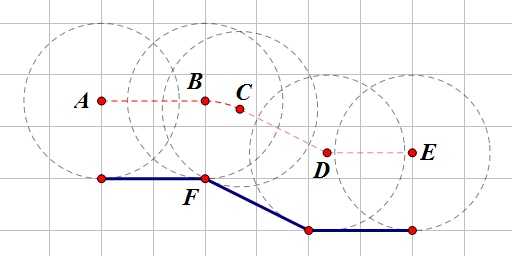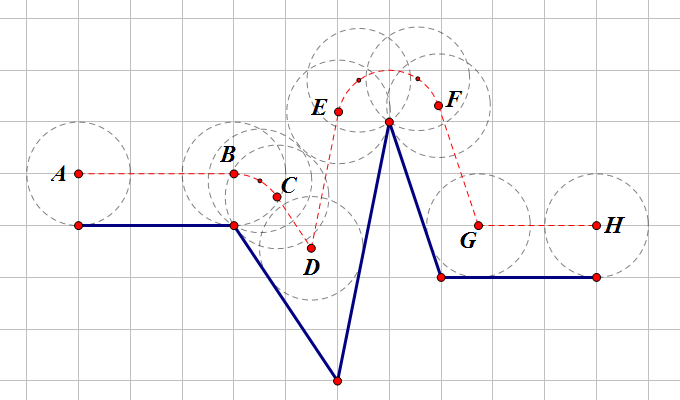×

# 计蒜客

1. 题库
2. 车轮轴迹
3. 问答
• 4.35%
• 65536K1 <= T <= 10

4 <= n <= 1000

0.5 <= r <= 20.0

0.0 <= \$x_i\$ <= 10000.0

-10000.0 <= \$y_i\$ <= 10000.0

```2
4 1.50
0.00 0.00
2.00 0.00
4.00 -1.00
6.00 -1.00
6 1.00
0.00 0.00
3.00 0.00
5.00 -3.00
6.00 2.00
7.00 -1.00
10.00 -1.00```

```6.22
15.20```

• main.c